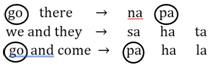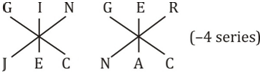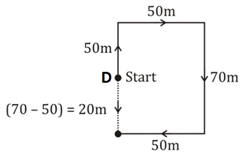# SSC Reasoning Ability Practice Questions (Day-61)

Dear Aspirants, The SSC Exams are going on at national level. Millions of candidates have eagerly prepared for the ongoing SSC exams such as CGL, CHSL, MTS, CPO, JE etc. SSC exams are usually conducted by the Staff Selection Commission to recruit the eligible candidates for various Central government departments. Mostly the selection process for all the SSC exams consists of the single or double phase of written examination based on the examination. Due to that, the written exam consisted of a certain syllabus and exam pattern. By following those regulations, the written exam has been conducted through an online mode. Based on the latest updated exam pattern and syllabus, here we have drafted the Reasoning Ability questions on a daily basis. So aspirants start your practice session with us and mould your skill in a perfect shape.

Start Quiz

1) Among four friends, Govind is twice the age of Krishan. Nandu is half of the age of Krishan. Gwala is 6 years elder to Krishan. Who is the youngest?

(a) Govind

(b) Krishan

(c) Nandu

(d) Cannot be determined

Direction (2-3): In the following question, select the word which cannot be formed using the letters of the given word.

2) Gregarious

(a) Vigour

(b) Aries

(c) Raser

(d) Sugar

3) Literature

(a) Literate

(b) Real

(c) Tile

(d) React

4) In a certain code language, “go there” is written as “na pa”, “we and they” is written as “sa ha ta” and “go and come” is written as “pa ha la”. How is “there”written in that code language?

(a) pa

(b) ha

(c) na

(d) la

5) In a certain code language, “RESIST” is written as “OANPOE” and “DIGENE” is written as “CEZAJA”. How is “GINGER” written in that code language?

(a) NACJEC

(b) KHFOBD

(c) MHFQDF

(d) JECNAC

6) In the following question, by using which mathematical operators will the expression become correct?

32 ?2 ?8 ?14 ? 4

(a) x, +, = and ÷

(b) +, +, = and x

(c) x, –, = and x

(d) +, x, = and x

7) In the following question, correct the equation by interchanging two signs.

7 × 6 + 5 – 12 ÷ 3 = 41

(a) + and ÷

(b) × and ÷

(c) + and –

(d) × and –

8) If 15 (196) 29 and 16 (100) 6, then what is the value of ‘A’ in 31 (A) 48?

(a) 361

(b) 256

(c) 324

(d) 289

9) If 72 A 7 B 9 = 16 and 52 A 5 B 7 = 12, then 92 A 3 B 8 = ?

(a) 17

(b) 92

(c) 86

(d) 35

10) From a point D Sarita walked 50 metres to the north, then after turning to right walked 50 metres. She again turned right and walked another 70 metres andﬁnally turned to right and walked 50 metres. In which direction and at what distance is she from the starting point D?

(a) 20 metres to the North

(b) 40 metres to the South

(c) 20 metres to the South

(d) 30 metres to the South

Govind = 2 × Krishan

Nandu = ½ × Krishan

Gwala = Krishan + 6

⇒ Nandu is the youngest.

‘Vigour’ ; ‘V’ is not present in the given word ‘Gregarious’.

‘React’ ; ‘C’ is not present in the given word.∴ There → na32 × 2 – 8 = 14 × 4

⇒ 64 – 8 = 56

⇒ 56 = 56

7 ×  6 + 5 – 12 ÷ 3 = 41

⇒ 7 × 6 –  5 + 12 ÷ 3 = 41

⇒ 42 – 5 + 4 = 41

⇒ 46 – 5 = 41

⇒ 41 = 41

29 – 15= 14  ; 142 = 196

16 – 6 = 10  ; 102 =  100

48 – 31 = 17 ; 172 = 289

72 ÷ 7 + 9 = 16

52 ÷ 5 + 7 = 12

92 ÷  3 + 8 = 35